Multiple Choice
Identify the choice that best completes the statement or answers the question.

1.

Algebraic Concepts – Patterns, Sequences, Functions – RIT 211 – 220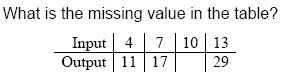a. 24 d. 13 b. 23 e. 25 c. 15

2.

Algebraic Concepts – Solving Equations & Inequalities – RIT 211 – 220

Solve:   x - 2.3 = 7.2
 a. x = 4.9 d. x = 9.23 b. x = 5.1 e. x = 95 c. x = 9.5

3.

Solve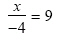a.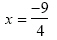d.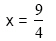b.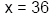e. x = -36 c.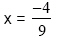4.

Solve:  x = 29 - 3(9 - 4)
 a. x = 130 d. x = -10 b. x = 14 e. x = -2 c. x = 6

5.

Algebraic Concepts – Patterns, Sequences, Functions – RIT 221 – 230

X         Y
6
9
5
2

Give the values for y in the given function:  y = x - 2
 a. 8, 11, 7, 4 d. 4, 7, 3, 2 b. 4, 7, 3, 0 e. 3, 4, 3, 1 c. 4, 7, 0, 2

6.

1, 2, 3, 5, 8, ____, _____, _____

Give the next three numbers in the pattern.
 a. 12, 17, 2 d. 13, 21, 34 b. 5, 3, 2 e. 11, 15, 20 c. 10, 12, 13

7.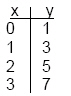What is the rule for this pattern?
 a.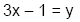d. 2x + 1 = y b.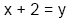e.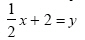c.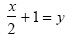8.

x + 1, x + 4, x + 9, x + 16, _____,  _____,  ______

Give the next three numbers in the sequence.
 a. x + 19, x + 22, x + 27 d. x + 23, x + 30, x + 37 b. x + 25, x + 36, x + 49 e. x + 17, x + 18, x + 19 c. x + 21, x + 24, x + 29

9.

Algebraic Concepts – Solving Equations & Inequalities – RIT 221 – 230

2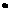+ 7 = 37
 a. 0 d. 10 b. 15 e. 12 c. 20

10.

Solve 6y  =  54
 a. y = 6 d. y = 7 b. y = 6 e. y = 9 c. y = 12

11.

Solve. X – (-6) = 4
 a. x = 2 d. x = -10 b. x = -2 e. x = 24 c. x = 10

12.

Solve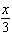+ 8 = - 2
 a. x = -30 d. x = 30 b. x = 14 e. x = 18 c. x = -14

13.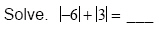a. –3 d. 9 b. –9 e. –2 c. 2

14.

Solve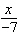=  - 3
 a. x = - 21 d. x = -b. x = -e. x = 4 c. x = 21

15.

Simplify. 3(x – 4)
 a. 3x + 12 d. 3x + 7 b. 3x – 12 e. x + 7 c. 3x – 7

16.

Computation – Decimals – RIT 211 – 220

Round 37.672 to the nearest whole number.
 a. 37.7 d. 37.6 b. 37 e. 38.6 c. 38

17.

Write 0.278 as a fraction.
 a. 278/100 d. 278/1,000 b. 2 78/100 e. 27 8/10 c. 278/10,000

18.

Round 3.5555 to the nearest thousandth.
 a. 3.6 d. 3.55 b. 4 e. 3.556 c. 3.56

19.

Use a calculator to find the sum.
0.3621 + 0.4795 =
 a. 0.8416 d. 84.16 b. 0.1174 e. .7396 c. 8.416

20.

0.35 x 0.43 =
 a. 0.78 d. 1.563 b. 0.1505 e. 1.5063 c. 0.156

21.

0.328  = _____
 a. 4.3 d. 280 b. 0.15 e. 43.75 c. 0.04